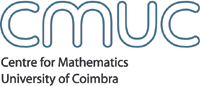AnalysisMembersSeminarsEventsPublicationsdetails Title Entropy numbers of embeddings of weighted Besov spaces. The limiting case. Abstract See here in pdf format The talk presents results of joint work with T.Kühn (Leipzig), W. Sickel (Jena) and L. Skrzypczak (Poznan). We investigate the asymptotic behaviour of the entropy numbers of the compact embedding id:B^{s_1}_{p_1,q_1}(\R^d, w) -----> B^{s_2}_{p_2,q_2}\,(\R^d) of the weighted Besov space B^{s_1}_{p_1,q_1}(\R^d, w) into the unweighted space B^{s_2}_{p_2,q_2}(\R^d). The weights which are admissible in our treatment are smooth, strictly positive, and satisfy \lim_{|x|\to \infty} w(x)= \infty. Most important for us will be the choice w_\alpha (x) = (1+ |x|^2)^{\alpha/2} for some \alpha >0. In the so called limiting situation, i.e. \alpha = \big(s_1-\frac{d}{p_1}\big) - \big(s_2-\frac{d}{p_2}\big) > \max{\big(0,\frac{d}{p_2} - \frac{d}{p_1}\big)} we give in almost all cases a sharp two-sided estimate. Speaker(s) Hans-Gerd Leopold (Friedrich-Schiller-University of Jena/Germany) Date September 24, 2004Time14h30 Room Room 5.5 CMUC Apartado 3008, 3001 - 454 Coimbra, Portugal T:+351 239 791 150 F:+351 239 793 069 cmuc@mat.uc.pt - developed by Flor de Utopia